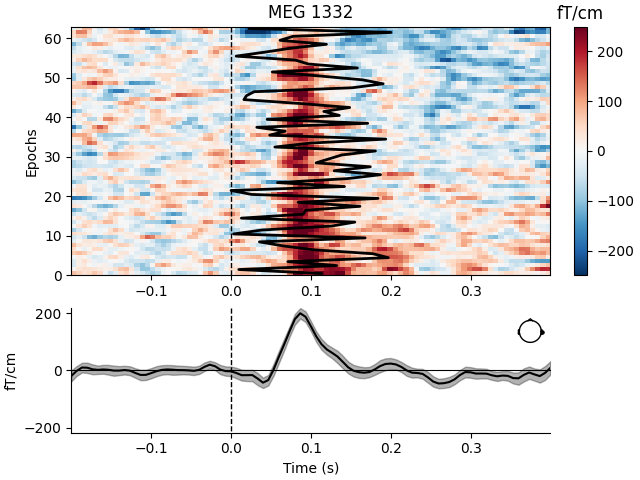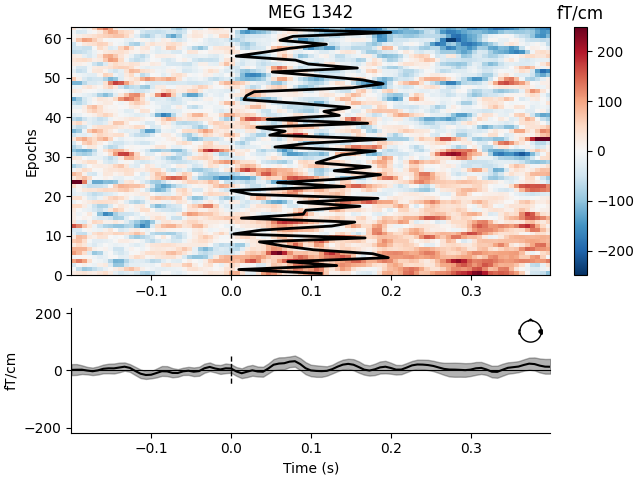# Visualize channel over epochs as an image#

This will produce what is sometimes called an event related potential / field (ERP/ERF) image.

Two images are produced, one with a good channel and one with a channel that does not show any evoked field.

It is also demonstrated how to reorder the epochs using a 1D spectral embedding as described in 1.

```# Authors: Alexandre Gramfort <alexandre.gramfort@inria.fr>
#
```
```import numpy as np
import matplotlib.pyplot as plt

import mne
from mne import io
from mne.datasets import sample

print(__doc__)

data_path = sample.data_path()
```

Set parameters

```meg_path = data_path / "MEG" / "sample"
raw_fname = meg_path / "sample_audvis_filt-0-40_raw.fif"
event_fname = meg_path / "sample_audvis_filt-0-40_raw-eve.fif"
event_id, tmin, tmax = 1, -0.2, 0.4

# Setup for reading the raw data

# Set up pick list: EEG + MEG - bad channels (modify to your needs)
raw.info["bads"] = ["MEG 2443", "EEG 053"]

# Create epochs, here for gradiometers + EOG only for simplicity
epochs = mne.Epochs(
raw,
events,
event_id,
tmin,
tmax,
proj=True,
baseline=(None, 0),
)
```
```Opening raw data file /home/circleci/mne_data/MNE-sample-data/MEG/sample/sample_audvis_filt-0-40_raw.fif...
Read a total of 4 projection items:
PCA-v1 (1 x 102)  idle
PCA-v2 (1 x 102)  idle
PCA-v3 (1 x 102)  idle
Average EEG reference (1 x 60)  idle
Range : 6450 ... 48149 =     42.956 ...   320.665 secs
72 matching events found
Setting baseline interval to [-0.19979521315838786, 0.0] s
Applying baseline correction (mode: mean)
4 projection items activated
Rejecting  epoch based on EOG : ['EOG 061']
Rejecting  epoch based on EOG : ['EOG 061']
Rejecting  epoch based on EOG : ['EOG 061']
Rejecting  epoch based on EOG : ['EOG 061']
Rejecting  epoch based on EOG : ['EOG 061']
Rejecting  epoch based on EOG : ['EOG 061']
Rejecting  epoch based on EOG : ['EOG 061']
Rejecting  epoch based on EOG : ['EOG 061']
Rejecting  epoch based on EOG : ['EOG 061']
```

Show event-related fields images

```# and order with spectral reordering
# If you don't have scikit-learn installed set order_func to None
from sklearn.manifold import spectral_embedding  # noqa
from sklearn.metrics.pairwise import rbf_kernel  # noqa

def order_func(times, data):
this_data = data[:, (times > 0.0) & (times < 0.350)]
this_data /= np.sqrt(np.sum(this_data**2, axis=1))[:, np.newaxis]
return np.argsort(
spectral_embedding(
rbf_kernel(this_data, gamma=1.0), n_components=1, random_state=0
).ravel()
)

good_pick = 97  # channel with a clear evoked response
bad_pick = 98  # channel with no evoked response

# We'll also plot a sample time onset for each trial
plt_times = np.linspace(0, 0.2, len(epochs))

plt.close("all")
mne.viz.plot_epochs_image(
epochs,
sigma=0.5,
order=order_func,
vmin=-250,
vmax=250,
overlay_times=plt_times,
show=True,
)
```
••```Not setting metadata
63 matching events found
No baseline correction applied
0 projection items activated